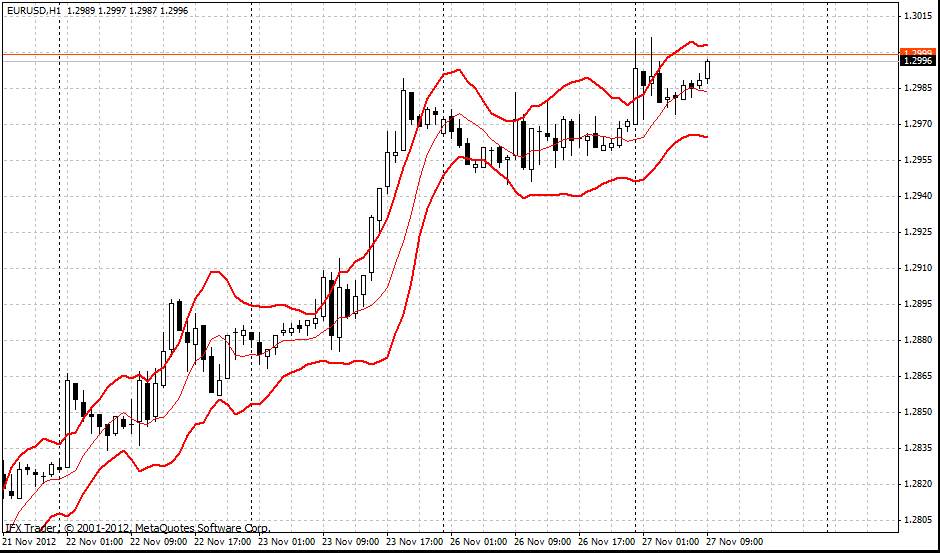# 斯塔克带指标

#### 公式

Median band = SMA(n) = SMA(5)

Upper band = SMA(n) + (ATR(m)*K) = SMA(5) + (ATR(15)*1,33)

Lower band = SMA(n) - (ATR(m)*K) = SMA(5) - (ATR(15)*1,33)

#### 交易使用#### InstaForex斯塔克带参数

BandsPeriod = 6

ATR = 10

K = 1.33

BandsShift = 2